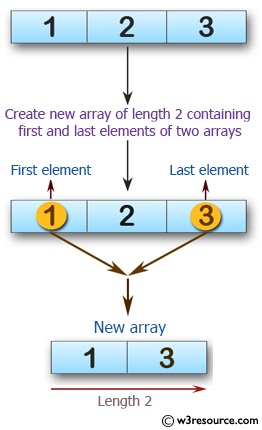﻿ Swift Array Programming Exercise: Create a new array of length 2 containing the first and last elements from a given array of integers. The given array length must be 1 or more - w3resource# Swift Array Programming Exercises: Create a new array of length 2 containing the first and last elements from a given array of integers. The given array length must be 1 or more

## Swift Array Programming: Exercise-10 with Solution

Write a Swift program to create a new array of length 2 containing the first and last elements from a given array of integers. The given array length must be 1 or more.

Pictorial Presentation:Sample Solution:

Swift Code:

``````func make_array(_ a: [Int]) -> [Int] {
var new_array: [Int] = []

new_array.append(a)
new_array.append(a.last!)

return new_array
}

print(make_array([1, 2, 3]))
print(make_array([1, 2, 3, 4]))
print(make_array([10, 11, 12, -10]))
```
```

Sample Output:

```[1, 3]
[1, 4]
[10, -10]
```

Swift Programming Code Editor:

Improve this sample solution and post your code through Disqus

What is the difficulty level of this exercise?

﻿